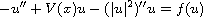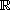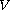Electron. J. Diff. Equ., Vol. 2015 (2015), No. 91, pp. 1-9.

### Existence of solutions to quasilinear Schrodinger equations with indefinite potential Zupei Shen, Zhiqing Han

Abstract:
In this article, we study the existence and multiplicity of solutions of the quasilinear Schrodinger equationon, where the potentialallows sign changing and the nonlinearity satisfies conditions weaker than the classical Ambrosetti-Rabinowitz condition. By a local linking theorem and the fountain theorem, we obtain the existence and multiplicity of solutions for the equation.

Submitted October 24, 2014. Published April 10, 2015.
Math Subject Classifications: 37J45, 58E05, 34C37, 70H05.
Key Words: Quasilinear Schrodinger equation; local linking; fountain theorem; indefinite potential.

Show me the PDF file (217 KB), TEX file, and other files for this article.Zupei Shen School of Mathematical Sciences Dalian University of Technology Dalian 116024, China email: pershen@mail.dlut.edu.cn Zhiqing Han School of Mathematical Sciences Dalian University of Technology Dalian 116024, China email: hanzhiq@dlut.edu.cn Phone(Fax) +86 41184707268